library(dimensio)

## Do PCA

## Load data
data(iris)
#>   Sepal.Length Sepal.Width Petal.Length Petal.Width Species
#> 1          5.1         3.5          1.4         0.2  setosa
#> 2          4.9         3.0          1.4         0.2  setosa
#> 3          4.7         3.2          1.3         0.2  setosa
#> 4          4.6         3.1          1.5         0.2  setosa
#> 5          5.0         3.6          1.4         0.2  setosa
#> 6          5.4         3.9          1.7         0.4  setosa

## Compute PCA
## (non numeric variables are automatically removed)
X <- pca(iris, center = TRUE, scale = TRUE)
#> 1 qualitative variable was removed: Species.

## Explore the results

dimensio provides several methods to extract (get_*()) the results:

• get_data() returns the original data.
• get_contributions() returns the contributions to the definition of the principal dimensions.
• get_coordinates() returns the principal or standard coordinates.
• get_correlations() returns the correlations between variables and dimensions.
• get_cos2() returns the cos2 values (i.e. the quality of the representation of the points on the factor map).
• get_eigenvalues() returns the eigenvalues, the percentages of variance and the cumulative percentages of variance.

The package also allows to quickly visualize (viz_*()) the results:

• biplot() produces a biplot.
• screeplot() produces a scree plot.
• viz_rows()/viz_individuals() displays row/individual principal coordinates.
• viz_columns()/viz_variables() displays columns/variable principal coordinates.
• viz_contributions() displays (joint) contributions.
• viz_cos2() displays (joint) cos2.
## Get eigenvalues
get_eigenvalues(X)
#>    eigenvalues  variance cumulative
#> F1   2.9184978 73.342264   73.34226
#> F2   0.9140305 22.969715   96.31198
#> F3   0.1467569  3.688021  100.00000

## Scree plot
screeplot(X, cumulative = TRUE)

## Plot variable contributions to the definition of the first two axes
viz_contributions(X, margin = 2, axes = c(1, 2))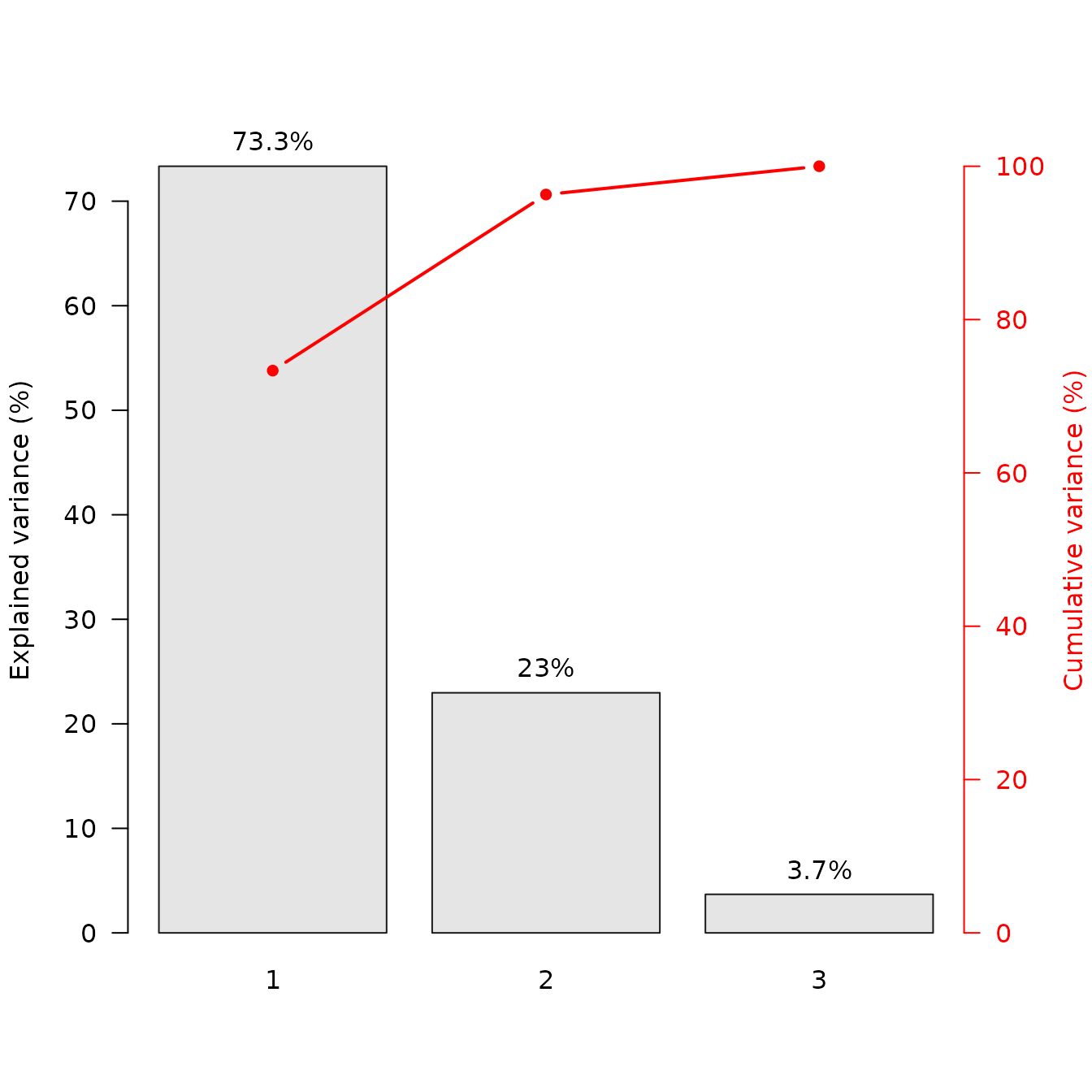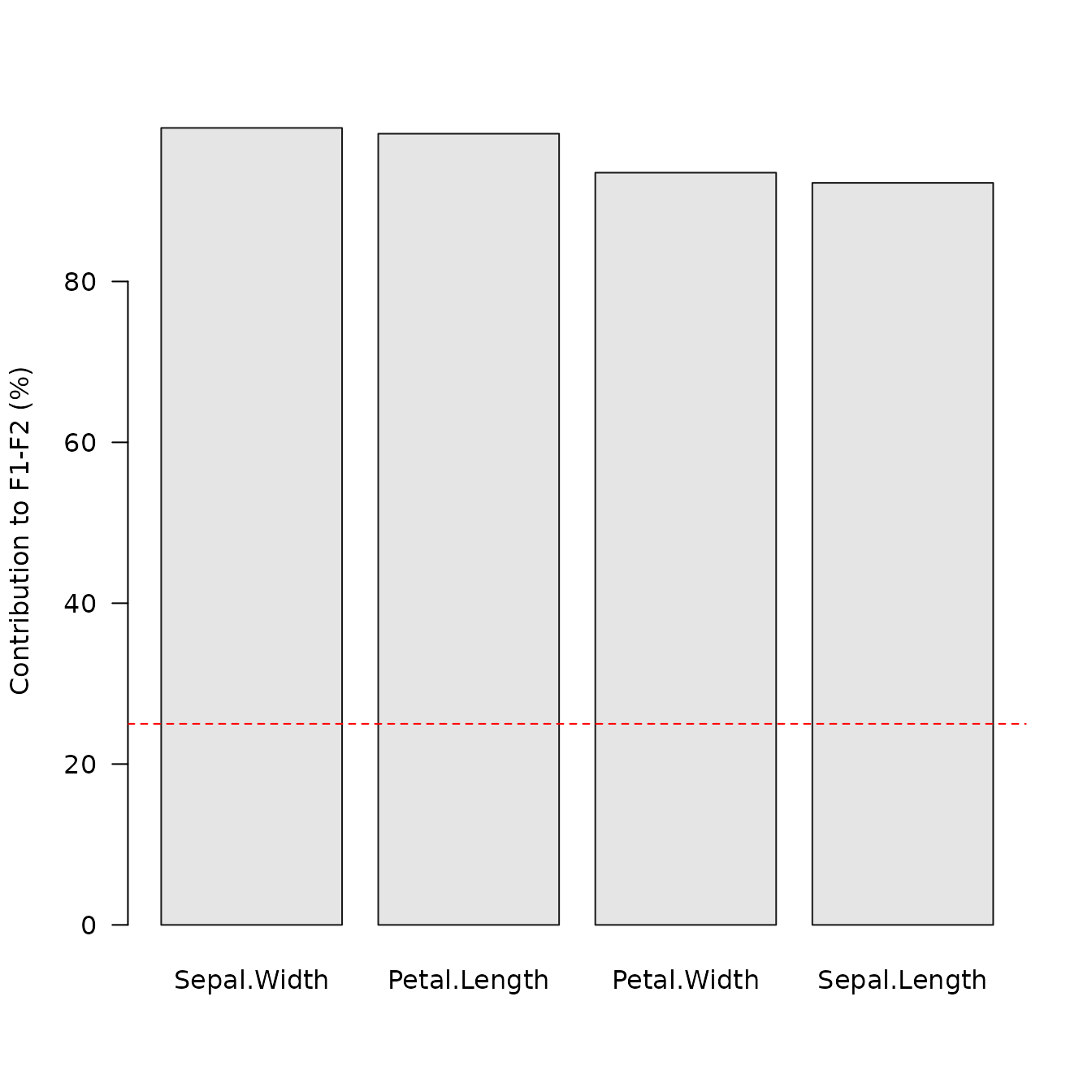## PCA biplot

A biplot is the simultaneous representation of rows and columns of a rectangular dataset. It is the generalization of a scatterplot to the case of mutlivariate data: it allows to visualize as much information as possible in a single graph (Greenacre 2010).

dimensio allows to display two types of biplots: a form biplot (row-metric-preserving biplot) or a covariance biplot (column-metric-preserving biplot). See Greenacre (2010) for more details about biplots.

The form biplot favors the representation of the individuals: the distance between the individuals approximates the Euclidean distance between rows. In the form biplot the length of a vector approximates the quality of the representation of the variable.

biplot(X, type = "form", label = "variables")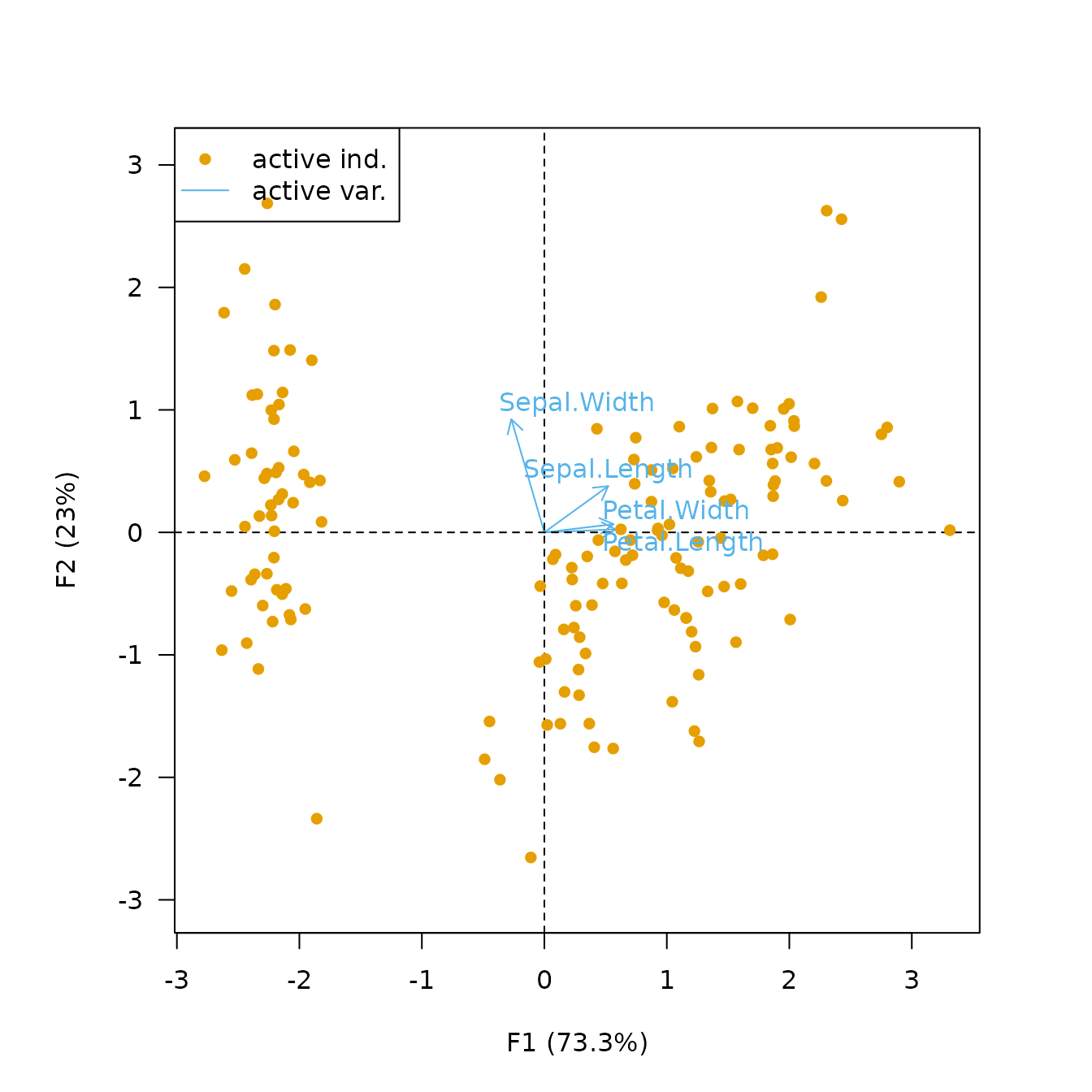The covariance biplot favors the representation of the variables: the length of a vector approximates the standard deviation of the variable and the cosine of the angle formed by two vectors approximates the correlation between the two variables (Greenacre 2010). In the covariance biplot the distance between the individuals approximates the Mahalanobis distance between rows.

biplot(X, type = "covariance", label = "variables")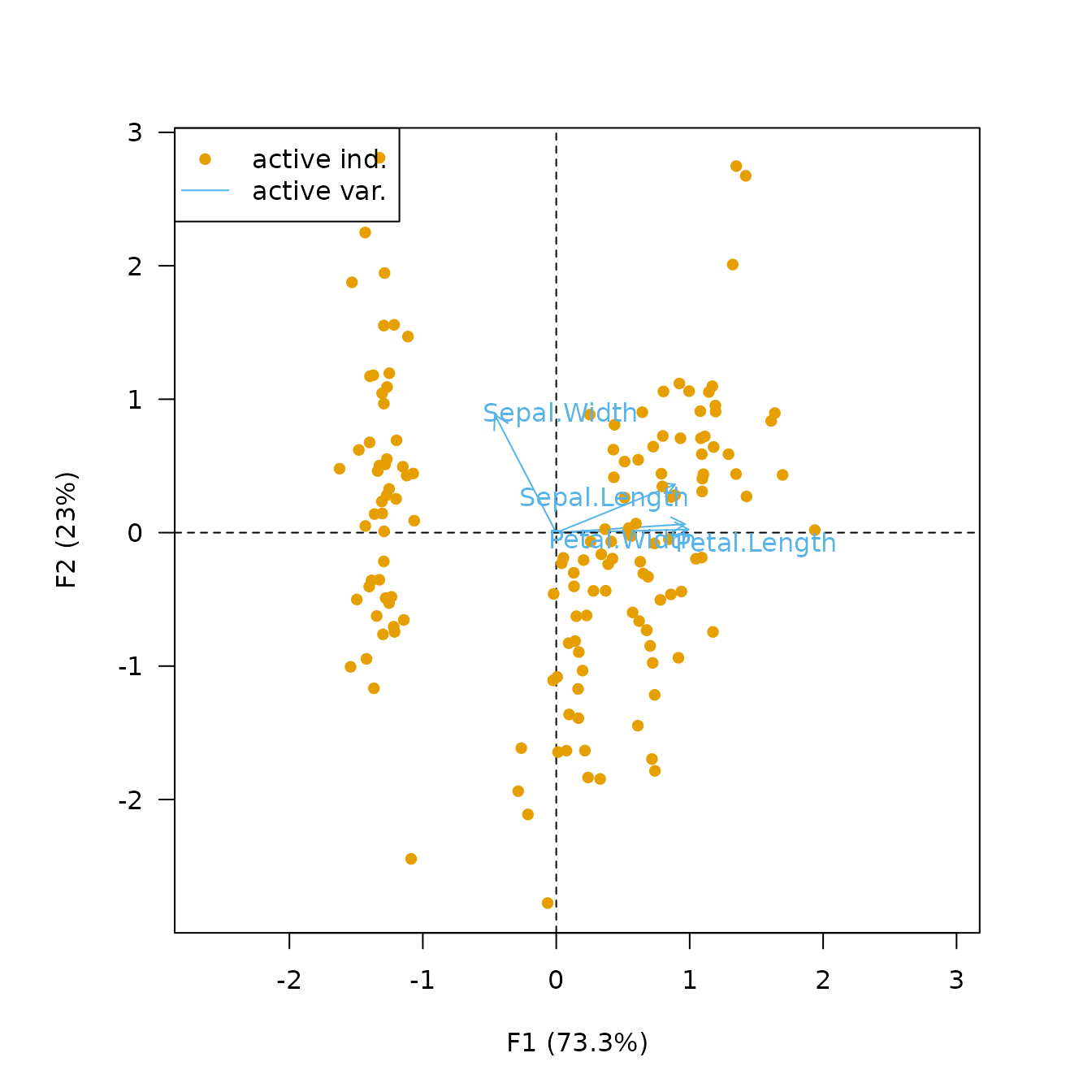Biplots have the drawbacks of their advantages: they can quickly become difficult to read as they display a lot of information at once. It may then be preferable to visualize the results for individuals and variables separately.

viz_variables() depicts the variables by rays emanating from the origin (both their lengths and directions are important to the interpretation).

## Plot variables factor map
viz_variables(X)viz_variables() allows to highlight additional information by varying different graphical elements (color, transparency, shape and size of symbols…).

## Highlight cos2
viz_variables(
x = X,
highlight = "cos2",
col = khroma::color("YlOrBr")(4, range = c(0.5, 1))
)## Plot PCA scores

viz_individuals() allows to display individuals and to highlight additional information.

## Plot individuals and color by species
viz_individuals(
x = X,
highlight = iris$Species, col = khroma::color("bright")(3), # Custom color scale pch = c(15, 16, 17) # Custom symbols )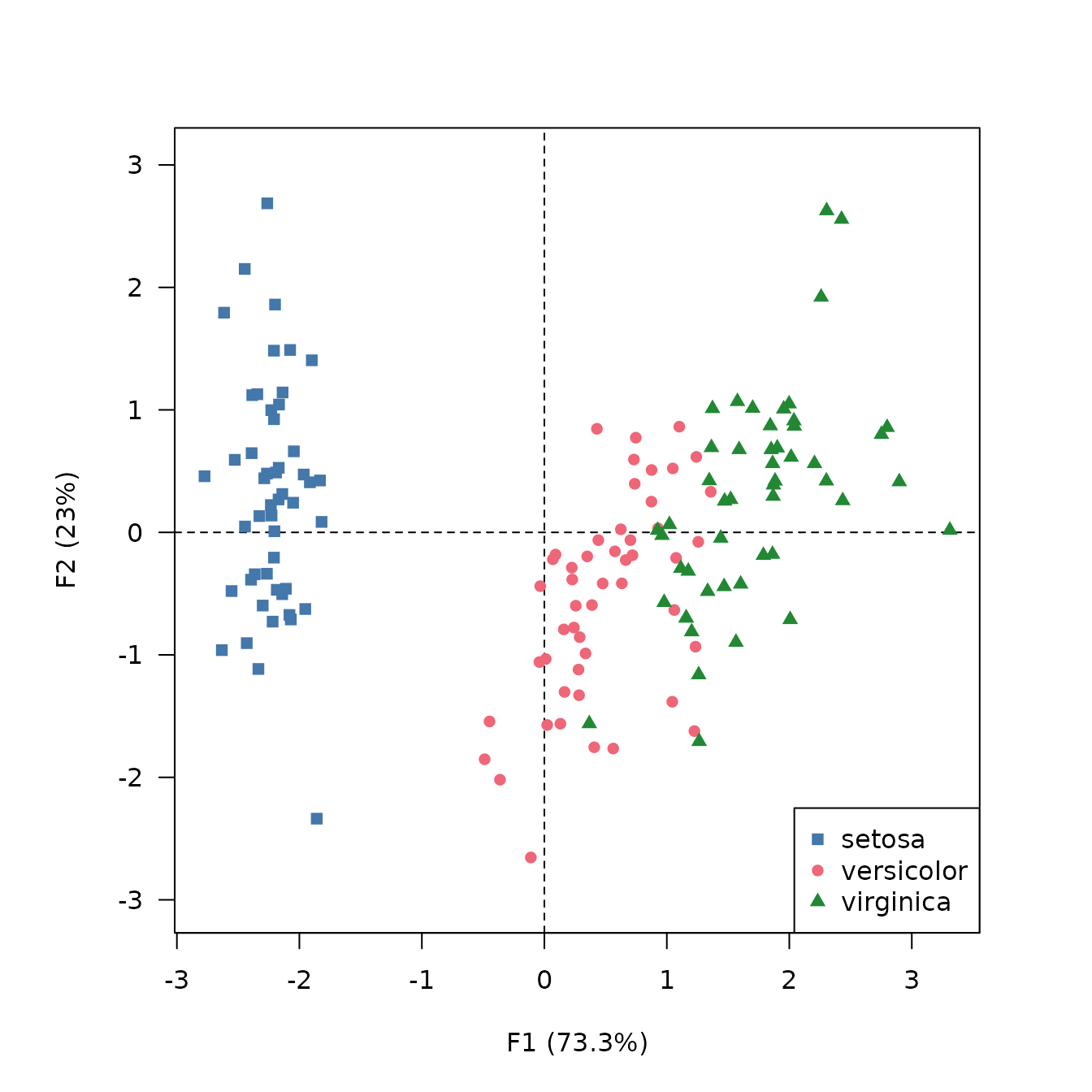## Add ellipses viz_individuals(x = X) viz_tolerance(x = X, group = iris$Species, level = 0.95,
border = khroma::color("high contrast")(3))

viz_individuals(x = X)
viz_hull(x = X, group = iris$Species, level = 0.95, border = khroma::color("high contrast")(3))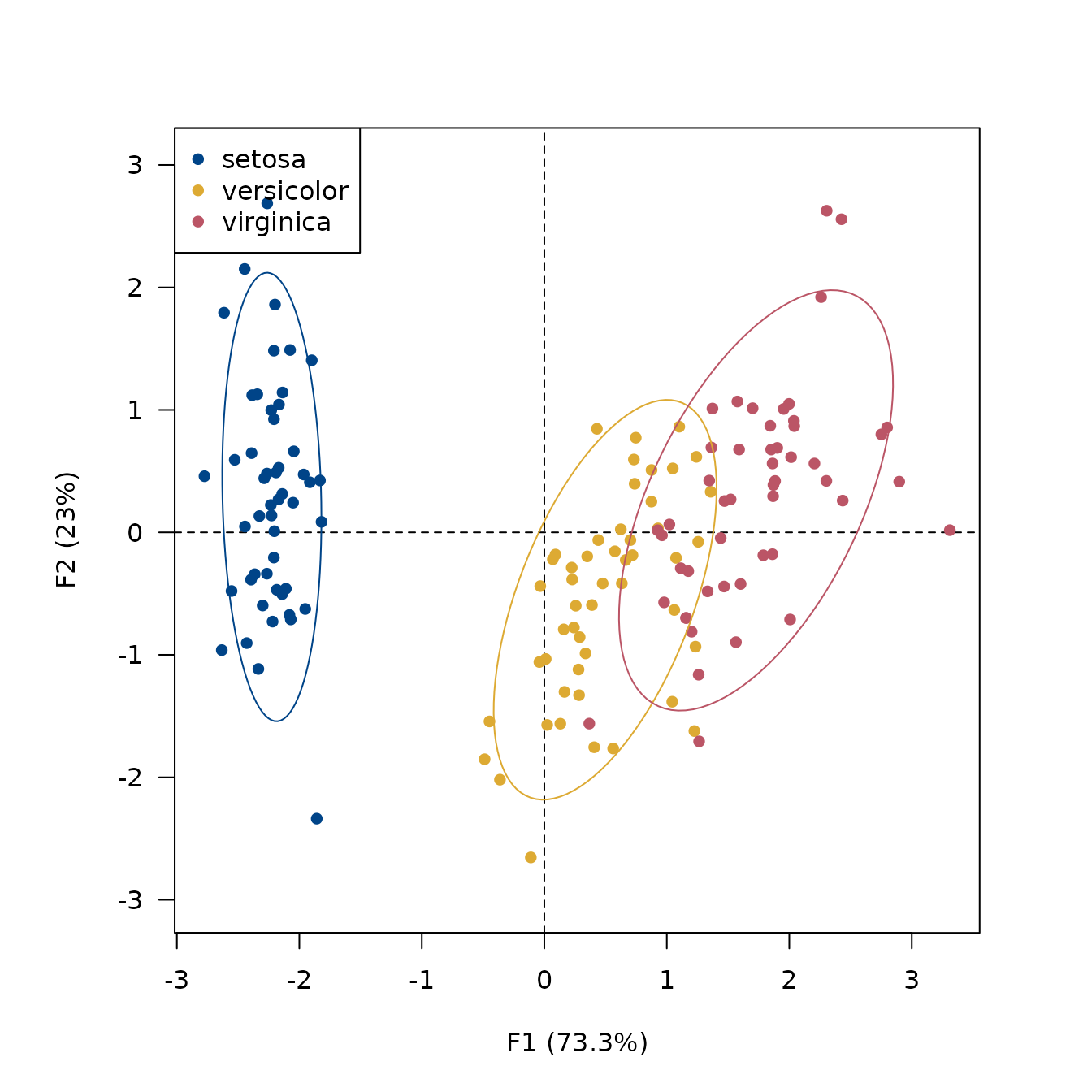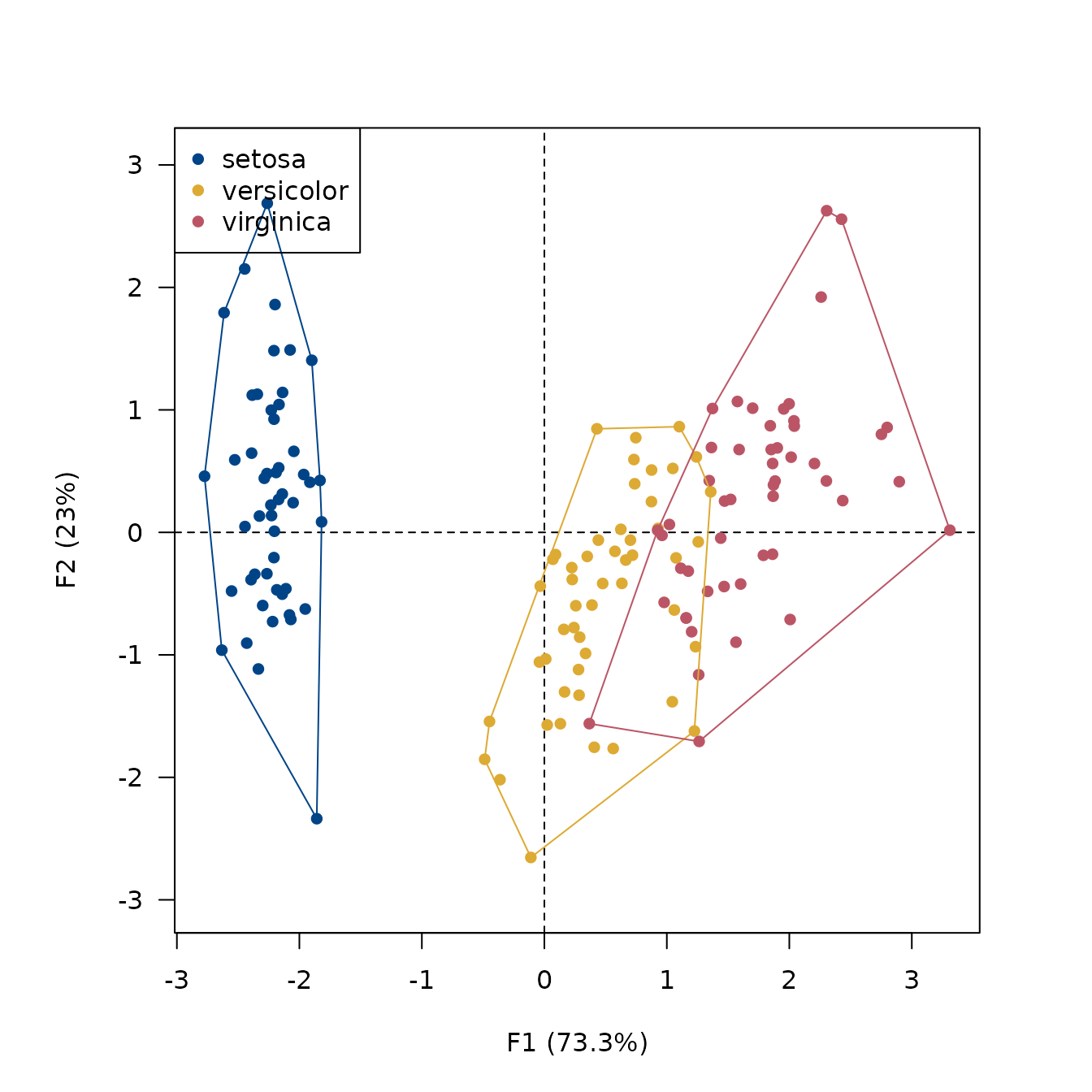## Highlight petal length viz_individuals( x = X, highlight = iris$Petal.Length,
col = khroma::color("YlOrBr")(12), # Custom color scale
cex = c(1, 2), # Custom size scale
pch = 16
)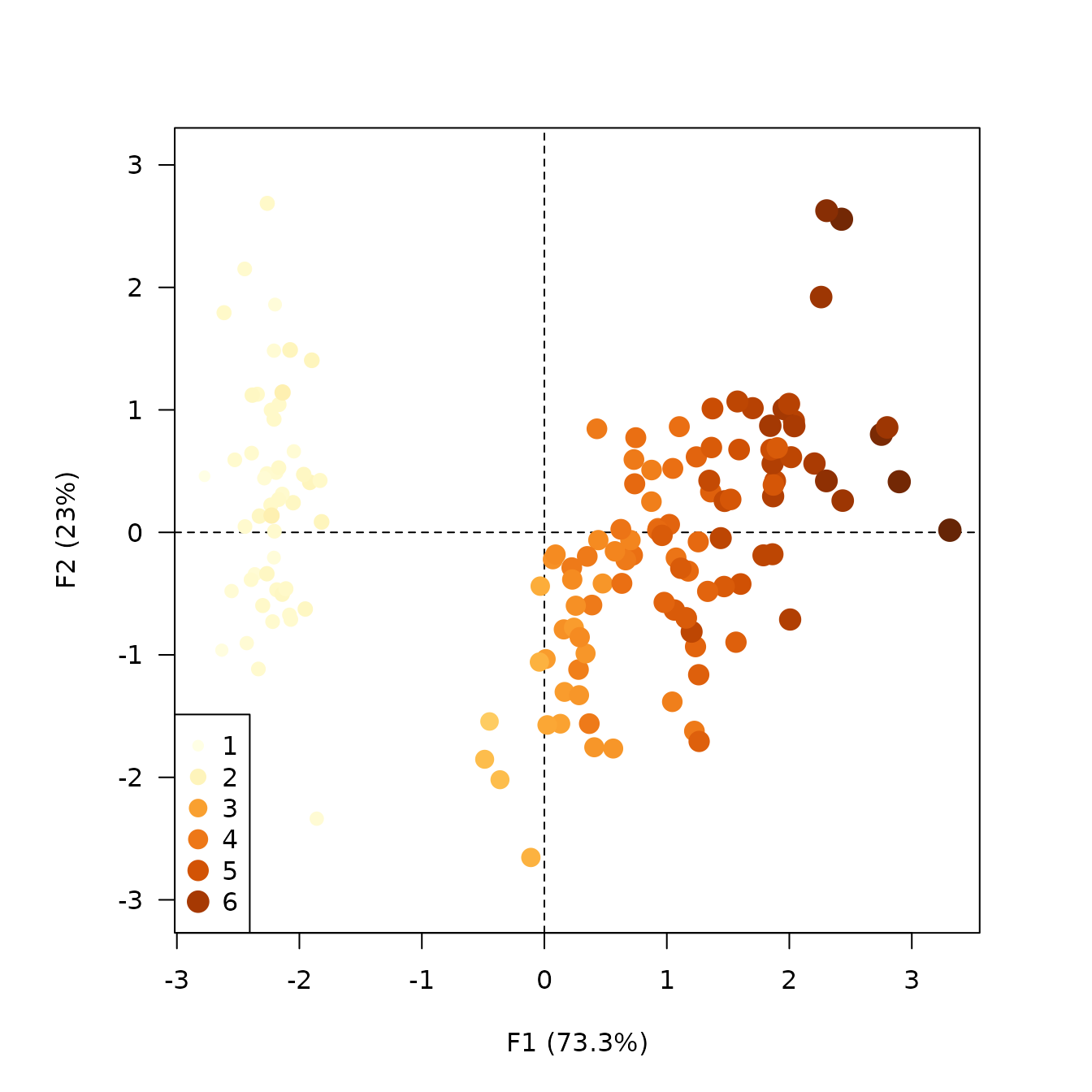## Highlight contributions
viz_individuals(
x = X,
highlight = "contrib",
col = khroma::color("iridescent")(12), # Custom color scale
cex = c(1, 2), # Custom size scale
pch = 16
)## Custom plot

If you need more flexibility, the get_*() family and the tidy() and augment() functions allow you to extract the results as data frames and thus build custom graphs with base graphics or ggplot2.

iris_tidy <- tidy(X, margin = 2)
#>          label component supplementary coordinate contribution        cos2
#> 1 Petal.Length        F1         FALSE 0.99155518  33.68793618 0.983181682
#> 2 Petal.Length        F2         FALSE 0.02341519   0.05998389 0.000548271
#> 3 Petal.Length        F3         FALSE 0.05444699   2.01999049 0.002964475
#> 4  Petal.Width        F1         FALSE 0.96497896  31.90629060 0.931184395
#> 5  Petal.Width        F2         FALSE 0.06399985   0.44812296 0.004095980
#> 6  Petal.Width        F3         FALSE 0.24298265  40.23019050 0.059040571

iris_augment <- augment(X, margin = 1)
#>          F1         F2 label supplementary        mass      sum contribution
#> 1 -2.264703  0.4800266     1         FALSE 0.006666667 5.359304     3.572870
#> 2 -2.080961 -0.6741336     2         FALSE 0.006666667 4.784855     3.189904
#> 3 -2.364229 -0.3419080     3         FALSE 0.006666667 5.706480     3.804320
#> 4 -2.299384 -0.5973945     4         FALSE 0.006666667 5.644048     3.762699
#> 5 -2.389842  0.6468354     5         FALSE 0.006666667 6.129742     4.086494
#> 6 -2.075631  1.4891775     6         FALSE 0.006666667 6.525894     4.350596
#>        cos2
#> 1 0.9968578
#> 2 0.9864650
#> 3 0.9995167
#> 4 0.9977577
#> 5 0.9997491
#> 6 0.9998819
## Custom plot with ggplot2
ggplot2::ggplot(data = iris_augment) +
ggplot2::aes(x = F1, y = F2, colour = contribution) +
ggplot2::geom_vline(xintercept = 0, linewidth = 0.5, linetype = "dashed") +
ggplot2::geom_hline(yintercept = 0, linewidth = 0.5, linetype = "dashed") +
ggplot2::geom_point() +
ggplot2::coord_fixed() + # /!\
ggplot2::theme_bw() +
khroma::scale_color_iridescent()
Greenacre, Michael J. 2010. Biplots in Practice. Bilbao: Fundación BBVA.